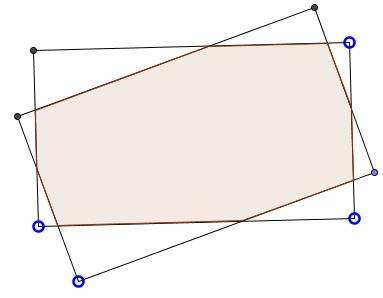# Area of the Intersection of Two Equal Rectangles

### Problem

This is Problem 15.47 from V. V. Prasolov, Problems in Geometry, V 2 (Nauka, 1986).

Two equal rectangles intersect in eight points.Prove that the area of their intersection (the common area, both of them enclose) is greater than half the area of any of them.

### Proof

Assume the rectangles are not square (this won't make much difference but will allow to talk of short and long sides - for convenience.) Having eight points of intersection means that each side of theirs intersects two sides of another rectangle - one long, one short. Let $A$ and $C$ be the intersections of the long sides, $B$ and $D$ the intersections of the short sides:We are going to prove that $AC\perp BD.$

Drop perpendiculars $CM$ and $CN$ on the opposite long sides. $CM=CN$ as both are equal the short side of the two rectangles. It follows that $\Delta ACM=\Delta ACN.$Thus $AC$ is the bisector of the angle between the two long sides. Similarly, $BD$ is the bisector of the angle between two short sides. They intersect, and are, therefore, perpendicular.We have $AC\times BD=2[ABCD],$ half the area of the quadrilateral $ABCD.$ Since one of them is not shorter than the short side, and the other is not shorter of the long side, of the rectangles, half their product is not less than half the area of each of the rectangles. But $ABCD$ is only a part of the common area enclosed by both rectangles. Thus the proof is complete.